# Tag: math

After the death of Grothendieck in November 2014, about 30.000 pages of his writings were found in Lasserre.Since then I’ve been trying to follow what happened to them:

So, what’s new?

Well, finally we have closure!

Last Friday, Grothendieck’s children donated the 30.000 Laserre pages to the Bibliotheque Nationale de France.

Via Des manuscrits inédits du génie des maths Grothendieck entrent à la BnF (and Google-translate):

“The singularity of these manuscripts is that they “cover many areas at the same time” to form “a whole, a + cathedral work +, with undeniable literary qualities”, analyzes Jocelyn Monchamp, curator in the manuscripts department of the BnF.

More than in “Récoltes et semailles”, very autobiographical, the author is “in a metaphysical retreat”, explains the curator, who has been going through the texts with passion for a month. A long-term task as the writing, in fountain pen, is dense and difficult to decipher. “I got used to it… And the advantage for us was that the author had methodically paginated and dated the texts.” One of the parts, entitled “Structures of the psyche”, a book of enigmatic diagrams translating psychology into algebraic language. In another, “The Problem of Evil”, he unfolds over 15,000 pages metaphysical meditations and thoughts on Satan. We sense a man “caught up by the ghosts of his past”, with an adolescence marked by the Shoah, underlines Johanna Grothendieck whose grandfather, a Russian Jew who fled Germany during the war, died at Auschwitz.

The deciphering work will take a long time to understand everything this genius wanted to say.

On Friday, the collection joined the manuscripts department of the Richelieu site, the historic cradle of the BnF, alongside the writings of Pierre and Marie Curie and Louis Pasteur. It will only be viewable by researchers.“This is a unique testimony in the history of science in the 20th century, of major importance for research,” believes Jocelyn Monchamp.

During the ceremony, one of the volumes was placed in a glass case next to a manuscript by the ancient Greek mathematician Euclid.”

Probably, the recent publication of Récoltes et Semailles clinched the deal.

Also, it is unclear at this moment whether the Istituto Grothendieck, which harbours The centre for Grothendieck studies coordinated by Mateo Carmona (see this post) played a role in the decision making, nor what role the Centre will play in the further studies of Grothendieck’s gribouillis.

For other coverage on this, see Hermit ‘scribblings’ of eccentric French math genius unveiled.

On the island of two truths, statements are either false (truth-value $0$), Q-true (value $Q$) or K-true (value $K$).

The King and Queen of the island have an opinion on all statements which may differ from their actual truth-value. We say that the Queen believes a statement $p$ is she assigns value $Q$ to it, and that she knows $p$ is she believes $p$ and the actual truth-value of $p$ is indeed $Q$. Similarly for the King, replacing $Q$’s by $K$’s.

All other inhabitants of the island are loyal to the Queen, or to the King, or to both. This means that they agree with the Queen (or King, or both) on all statements they have an opinion on. Two inhabitants are said to be loyal to each other if they agree on all statements they both have an opinion of.

Last time we saw that Queen and King agree on all statements one of them believes to be false, as well as the negation of such statements. This raised the question:

Are the King and Queen loyal to each other? That is, do Queen and King agree on all statements?

We cannot resolve this issue without the information Oscar was able to extract from Pointex in Karin Cvetko-Vah‘s post Pointex:

“Oscar was determined to get some more information. “Could you at least tell me whether the queen and the king know that they’re loyal to themselves?” he asked.
“Well, of course they know that!” replied Pointex.
“You said that a proposition can be Q-TRUE, K-TRUE or FALSE,” Oscar said.
“Yes, of course. What else!” replied Pointex as he threw the cap high up.
“Well, you also said that each native was loyal either to the queen or to the king. I was just wondering … Assume that A is loyal to the queen. Then what is the truth value of the statement: A is loyal to the queen?”
“Q, of course,” answered Pointex as he threw the cap up again.
“And what if A is not loyal to the queen? What is then the truth value of the statement: A is loyal to the queen?”
He barely finished his question as something fell over his face and covered his eyes. It was the funny cap.
“Thanx,” said Pointex as Oscar handed him the cap. “The value is 0, of course.”
“Can the truth value of the statement: ‘A is loyal to the queen’ be K in any case?”
“Well, what do you think? Of course not! What a ridiculous thing to ask!” replied Pointex.”

Puzzle : Show that Queen and King are not loyal to each other, that is, there are statements on which they do not agree.Solution : ‘The King is loyal to the Queen’ must have actual truth-value $0$ or $Q$, and the sentence ‘The Queen is loyal to the King’ must have actual truth-value $0$ or $K$. But both these sentences are the same as the sentence ‘The Queen and King are loyal to each other’ and as this sentence can have only one truth-value, it must have value $0$ so the statement is false.

Note that we didn’t produce a specific statement on which the Queen and King disagree. Can you find one?

Last time we had a brief encounter with the island of two truths, invented by Karin Cvetko-Vah. See her posts:

On this island, false statements have truth-value $0$ (as usual), but non-false statements are not necessarily true, but can be given either truth-value $Q$ (statements which the Queen on the island prefers) or $K$ (preferred by the King).

Think of the island as Trump’s paradise where nobody is ever able to say: “Look, alternative truths are not truths. They’re falsehoods.”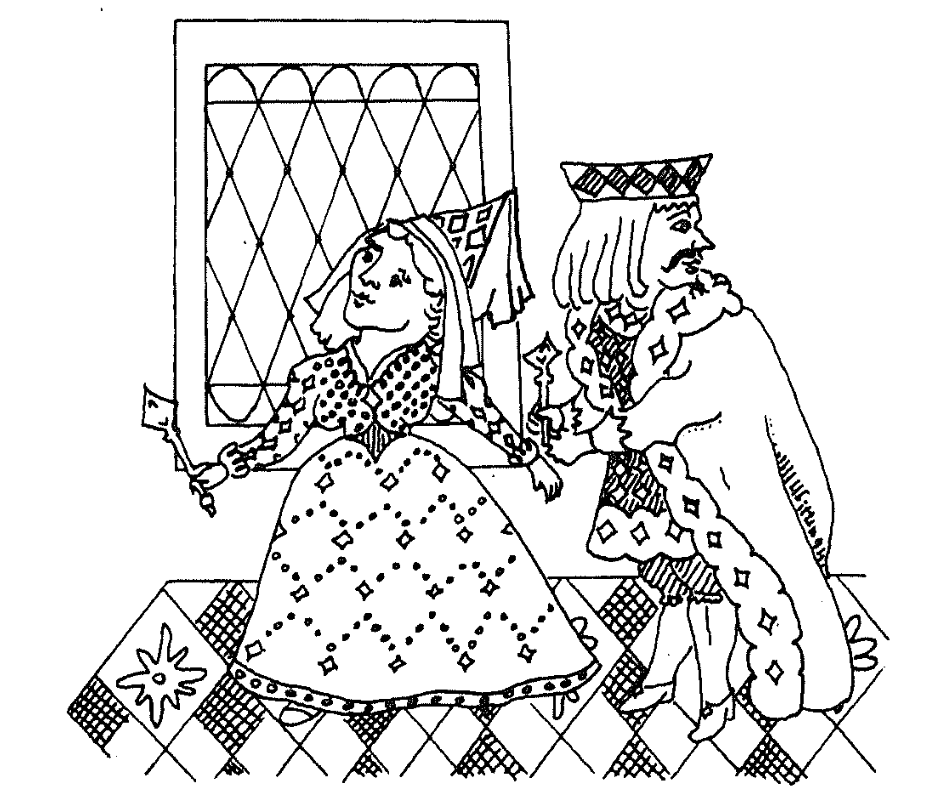Even the presence of just one ‘alternative truth’ has dramatic consequences on the rationality of your reasoning. If we know the truth-values of specific sentences, we can determine the truth-value of more complex sentences in which we use logical connectives such as $\vee$ (or), $\wedge$ (and), $\neg$ (not), and $\implies$ (then) via these truth tables:

$\begin{array}{c|ccc} \downarrow~\bf{\wedge}~\rightarrow & 0 & Q & K \\ \hline 0 & 0 & 0 & 0 \\ Q & 0 & Q & Q \\ K & 0 & K & K \end{array} \quad \begin{array}{c|ccc} \downarrow~\vee~\rightarrow & 0 & Q & K \\ \hline 0 & 0 & Q & K \\ Q & Q & Q & K \\ K & K & Q & K \end{array}$
$\begin{array}{c|ccc} \downarrow~\implies~\rightarrow & 0 & Q & K \\ \hline 0 & Q & Q & K \\ Q & 0 & Q & K \\ K & 0 & Q & K \end{array} \quad \begin{array}{c|c} \downarrow & \neg~\downarrow \\ \hline 0 & Q \\ Q & 0 \\ K & 0 \end{array}$

Note that the truth-values $Q$ and $K$ are not completely on equal footing as we have to make a choice which one of them will stand for $\neg 0$.

Common tautologies are no longer valid on this island. The best we can have are $Q$-tautologies (giving value $Q$ whatever the values of the components) or $K$-tautologies.

Here’s one $Q$-tautology (check!) : $(\neg p) \vee (\neg \neg p)$. Verify that $p \vee (\neg p)$ is neither a $Q$- nor a $K$-tautology.

Can you find any $K$-tautology at all?

Already this makes it incredibly difficult to adapt Smullyan-like Knights and Knaves puzzles to this skewed island. Last time I gave one easy example.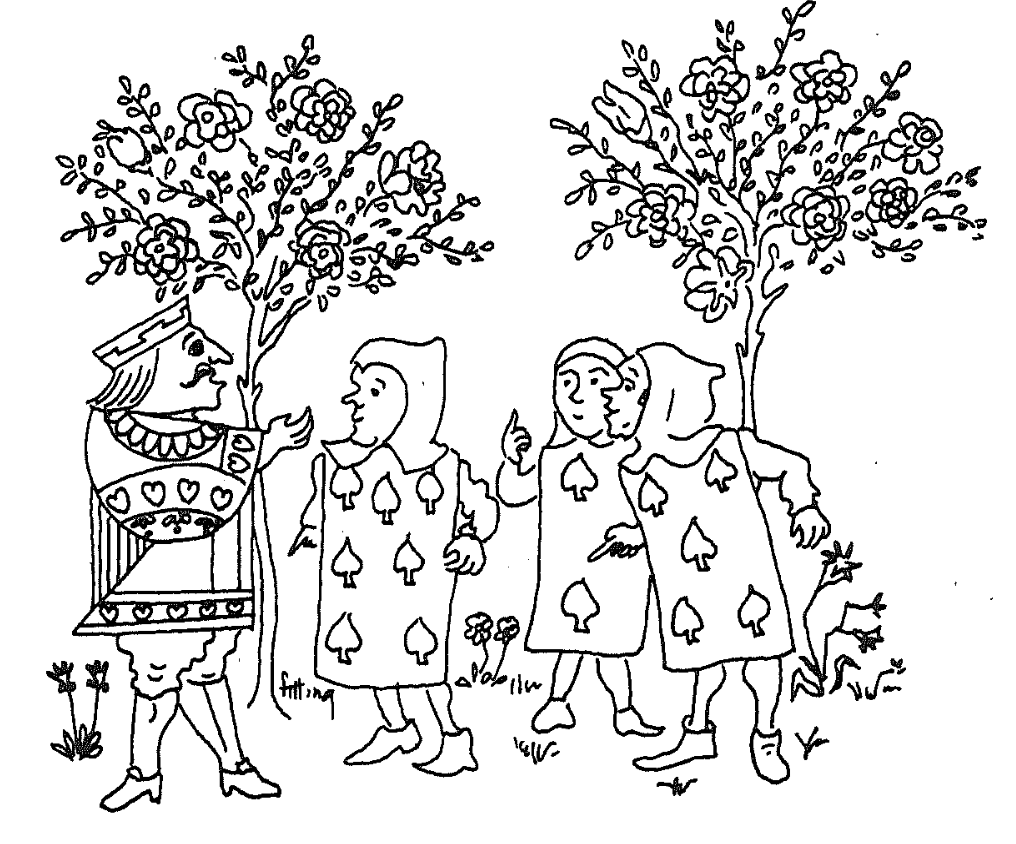Puzzle : On an island of two truths all inhabitants are either Knaves (saying only false statements), Q-Knights (saying only $Q$-valued statements) or K-Knights (who only say $K$-valued statements).

The King came across three inhabitants, whom we will call $A$, $B$ and $C$. He asked $A$: “Are you one of my Knights?” $A$ answered, but so indistinctly that the King could not understand what he said.

He then asked $B$: “What did he say?” $B$ replies: “He said that he is a Knave.” At this point, $C$ piped up and said: “That’s not true!”

Was $C$ a Knave, a Q-Knight or a K-Knight?

Solution : Q- and K-Knights can never claim to be a Knave. Neither can Knaves because they can only say false statements. So, no inhabitant on the island can ever claim to be a Knave. So, $B$ lies and is a Knave, so his stament has truth-value $0$. $C$ claims the negation of what $B$ says so the truth-value of his statement is $\neg 0 = Q$. $C$ must be a Q-Knight.

As if this were not difficult enough, Karin likes to complicate things by letting the Queen and King assign their own truth-values to all sentences, which may coincide with their actual truth-value or not.

Clearly, these two truth-assignments follow the logic of the island of two truths for composed sentences, and we impose one additional rule: if the Queen assigns value $0$ to a statement, then so does the King, and vice versa.

I guess she wanted to set the stage for variations to the island of two truths of epistemic modal logical puzzles as in Smullyan’s book Forever Undecided (for a quick summary, have a look at Smullyan’s paper Logicians who reason about themselves).

A possible interpretation of the Queen’s truth-assignment is that she assigns value $Q$ to all statements she believes to be true, value $0$ to all statements she believes to be false, and value $K$ to all statements she has no fixed opinion on (she neither believes them to be true nor false). The King assigns value $K$ to all statements he believes to be true, $0$ to those he believes to be false, and $Q$ to those he has no fixed opinion on.

For example, if the Queen has no fixed opinion on $p$ (so she assigns value $K$ to it), then the King can either believe $p$ (if he also assigns value $K$ to it) or can have no fixed opinion on $p$ (if he assigns value $Q$ to it), but he can never believe $p$ to be false.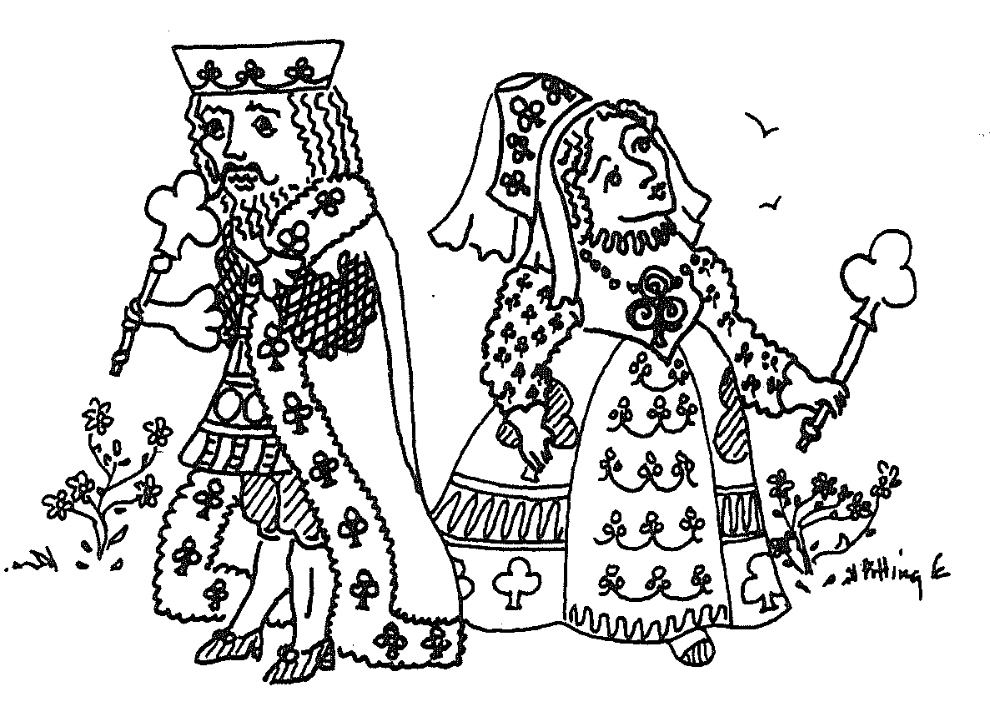Puzzle : We say that Queen and King ‘agree’ on a statement $p$ if they both assign the same value to it. So, they agree on all statements one of them (and hence both) believe to be false. But there’s more:

• Show that Queen and King agree on the negation of all statements one of them believes to be false.
• Show that the King never believes the negation of whatever statement.
• Show that the Queen believes all negations of statements the King believes to be false.

Solution : If one of them believes $p$ to be false (s)he will assign value $0$ to $p$ (and so does the other), but then they both have to assign value $Q$ to $\neg p$, so they agree on this.

The value of $\neg p$ can never be $K$, so the King does not believe $\neg p$.

If the King believes $p$ to be false he assigns value $0$ to it, and so does the Queen, but then the value of $\neg p$ is $Q$ and so the Queen believes $\neg p$.

We see that the Queen and King agree on a lot of statements, they agree on all statements one of them believes to be false, and they agree on the negation of such statements!

Can you find any statement at all on which they do not agree?

Well, that may be a little bit premature. We didn’t say which sentences about the island are allowed, and what the connection (if any) is between the Queen and King’s value-assignments and the actual truth values.

For example, the Queen and King may agree on a classical ($0$ or $1$) truth-assignments to the atomic sentences for the island, and replace all $1$’s with $Q$. This will give a consistent assignment of truth-values, compatible with the island’s strange logic. (We cannot do the same trick replacing $1$’s by $K$ because $\neg 0 = Q$).

Clearly, such a system may have no relation at all with the intended meaning of these sentences on the island (the actual truth-values).

That’s why Karin Cvetko-Vah introduced the notions of ‘loyalty’ and ‘sanity’ for inhabitants of the island. That’s for next time, and perhaps then you’ll be able to answer the question whether Queen and King agree on all statements.

(all images in this post are from Smullyan’s book Alice in Puzzle-Land)Raymond Smullyan‘s logic puzzles are legendary. Among his best known are his Knights (who always tell the truth) and Knaves (who always lie) puzzles. Here’s a classic example.

“On the day of his arrival, the anthropologist Edgar Abercrombie came across three inhabitants, whom we will call $A$, $B$ and $C$. He asked $A$: “Are you a Knight or a Knave?” $A$ answered, but so indistinctly that Abercrombie could not understand what he said.

He then asked $B$: “What did he say?” $B$ replies: “He said that he is a knave.” At this point, $C$ piped up and said: “Don’t believe that; it’s a lie!”

Was $C$ a Knight or a Knave?”

If you are stumped by this, try to figure out what kind of inhabitant can say “I am a Knave”.

Some years ago, my friend and co-author Karin Cvetko-Vah wrote about a much stranger island, the island of two truths.

“The island was ruled by a queen and a king. It is important to stress that the queen was neither inferior nor superior to the king. Rather than as a married couple one should think of the queen and the king as two parallel powers, somewhat like the Queen of the Night and the King Sarastro in Mozart’s famous opera The Magic Flute. The queen and the king had their own castle each, each of them had their own court, their own advisers and servants, and most importantly each of them even had their own truth value.

On the island, a proposition p is either FALSE, Q-TRUE or K-TRUE; in each of the cases we say that p has value 0, Q or K, respectively. The queen finds the truth value Q to be superior, while the king values the most the value K. The queen and the king have their opinions on all issues, while other residents typically have their opinions on some issues but not all.”

The logic of the island of two truths is the easiest example of what Karin and I called a non-commutative frame or skew Heyting algebra (see here), a notion we then used, jointly with Jens Hemelaer, to define the notion of a non-commutative topos.If you take our general definitions, and take Q as the distinguished top-element, then the truth tables for the island of two truths are these ones (value of first term on the left, that of the second on top):

$\begin{array}{c|ccc} \wedge & 0 & Q & K \\ \hline 0 & 0 & 0 & 0 \\ Q & 0 & Q & Q \\ K & 0 & K & K \end{array} \quad \begin{array}{c|ccc} \vee & 0 & Q & K \\ \hline 0 & 0 & Q & K \\ Q & Q & Q & K \\ K & K & Q & K \end{array} \quad \begin{array}{c|ccc} \rightarrow & 0 & Q & K \\ \hline 0 & Q & Q & K \\ Q & 0 & Q & K \\ K & 0 & Q & K \end{array} \quad \begin{array}{c|c} & \neg \\ \hline 0 & Q \\ Q & 0 \\ K & 0 \end{array}$

Note that on this island the order of statements is important! That is, the truth value of $p \wedge q$ may differ from that of $q \wedge p$ (and similarly for $\vee$).

Let’s reconsider Smullyan’s puzzle at the beginning of this post, but now on an island of two truths, where every inhabitant is either of Knave, or a Q-Knight (uttering only Q-valued statements), or a K-Knight (saying only K-valued statements).

Again, can you determine what type $C$ is?

Well, if you forget about the distinction between Q- and K-valued sentences, then we’re back to classical logic (or more generally, if you divide out Green’s equivalence relation from any skew Heyting algebra you obtain an ordinary Heyting algebra), and we have seen that then $B$ must be a Knave and $C$ a Knight, so in our new setting we know that $C$ is either a Q-Knight or a K-Knight, but which of the two?

Now, $C$ claims the negation of what $B$ said, so the truth value is $\neg 0 = Q$, and therefore $C$ must be a Q-Knight.

Recall that in Karin Cvetko-Vah‘s island of two truths all sentences have a unique value which can be either $0$ (false) or one of the non-false values Q or K, and the value of combined statements is given by the truth tables above. The Queen and King both have an opinion on all statements, which may or may not coincide with the actual value of that statement. However, if the Queen assigns value $0$ to a statement, then so does the King, and conversely.

Other inhabitants of the island have only their opinion about a subset of all statements (which may be empty). Two inhabitants agree on a statement if they both have an opinion on it and assign the same value to it.

Now, each inhabitant is either loyal to the Queen or to the King (or both), meaning that they agree with the Queen (resp. King) on all statements they have an opinion of. An inhabitant loyal to the Queen is said to believe a sentence when she assigns value $Q$ to it (and symmetric for those loyal to the King), and knows the statement if she believes it and that value coincides with the actual value of that statement.

Further, if A is loyal to the Queen, then the value of the statement ‘A is loyal to the Queen’ is Q, and if A is not loyal to the Queen, then the value of the sentence ‘A is loyal to the Queen’ is $0$ (and similarly for statements about loyalty to the King).

These notions are enough for the first batch of ten puzzles in Karin’s posts

Just one example:

Show that if anybody on the island knows that A is not loyal to the Queen, then everybody that has an opinion about the sentence ‘A is loyal to the Queen’ knows that.

After these two posts, Karin decided that it was more fun to blog about the use of non-commutative frames in data analysis.

But, she once gave me a text containing many more puzzles (as well as all the answers), so perhaps I’ll share these in a follow-up post.

A projective plane of order $n$ is a collection of $n^2+n+1$ lines and $n^2+n+1$ points satisfying:

• every line contains exactly $n+1$ points
• every point lies on exactly $n+1$ lines
• any two distinct lines meet at exactly one point
• any two distinct points lie on exactly one line

Clearly, if $q=p^k$ is a pure prime power, then the projective plane over $\mathbb{F}_q$, $\mathbb{P}^2(\mathbb{F}_q)$ (that is, all nonzero triples of elements from the finite field $\mathbb{F}_q$ up to simultaneous multiplication with a non-zero element from $\mathbb{F}_q$) is a projective plane of order $q$.

The easiest example being $\mathbb{P}^2(\mathbb{F}_2)$ consisting of seven points and lines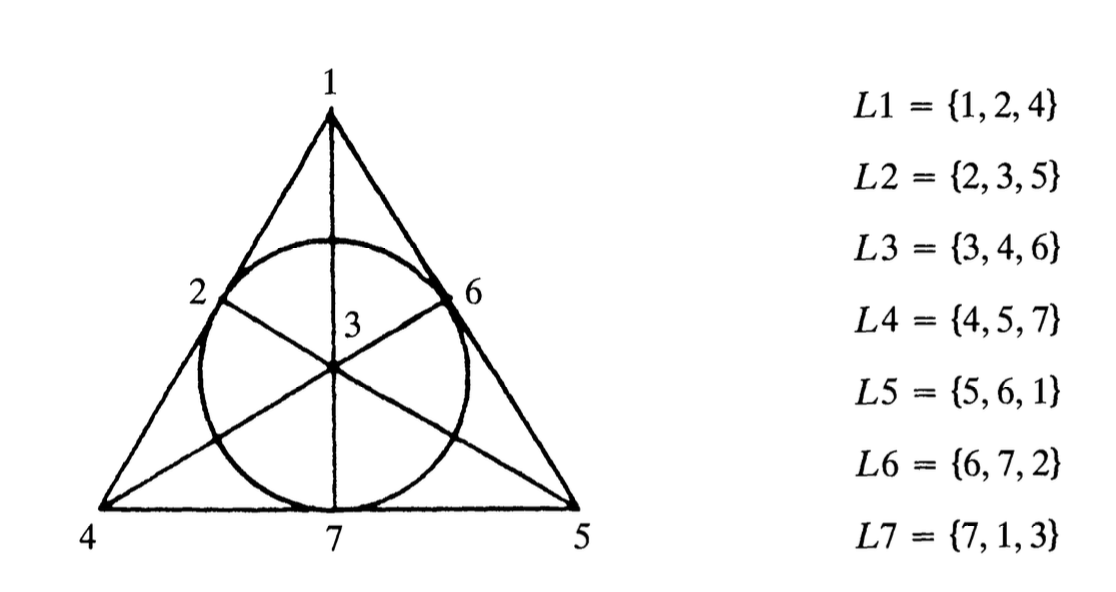But, there are others. A triangle is a projective plane of order $1$, which is not of the above form, unless you believe in the field with one element $\mathbb{F}_1$…

And, apart from $\mathbb{P}^2(\mathbb{F}_{3^2})$, there are three other, non-isomorphic, projective planes of order $9$.

It is clear then that for all $n < 10$, except perhaps $n=6$, a projective plane of order $n$ exists.

In 1938, Raj Chandra Bose showed that there is no plane of order $6$ as there cannot be $5$ mutually orthogonal Latin squares of order $6$, when even the problem of two orthogonal squares of order $6$ (see Euler’s problem of the $36$ officers) is impossible.

Yeah yeah Bob, I know it has a quantum solution.

Anyway by May 1977, when Lenstra’s Festschrift ‘Een pak met een korte broek’ (a suit with shorts) was published, the existence of a projective plane of order $10$ was still wide open.

That’s when Andrew Odlyzko (probably known best for his numerical work on the Riemann zeta function) and Neil Sloane (probably best known as the creator of the On-Line Encyclopedia of Integer Sequences) joined forces to publish in Lenstra’s festschrift a note claiming (jokingly) the existence of a projective plane of order ten, as they were able to find a finite field of ten elements.Here’s a transcript:

A PROJECTIVE PLAIN OF ORDER TEN

A. M. Odlyzko and N.J.A. Sloane

This note settles in the affirmative the notorious question of the existence of a projective plain of order ten.

It is well-known that if a finite field $F$ is given containing $n$ elements, then the projective plain of order $n$ can be immediately constructed (see M. Hall Jr., Combinatorial Theory, Blaisdell, Waltham, Mass. 1967 and D.R. Hughes and F.C. Piper, Projective Planes, Springer-Verlag, N.Y., 1970).

For example, the points of the plane are represented by the nonzero triples $(\alpha,\beta,\gamma)$ of elements of $F$, with the convention that $(\alpha,\beta,\gamma)$ and $(r\alpha, r\beta, r\gamma)$ represent the same point, for all nonzero $r \in F$.

Furthermore this plain even has the desirable property that Desargues’ theorem holds there.

What makes this note possible is our recent discovery of a field containing exactly ten elements: we call it the digital field.

We first show that this field exists, and then give a childishly simple construction which the reader can easily verify.

The Existence Proof

Since every real number can be written in the decimal system we conclude that

$\mathbb{R} = GF(10^{\omega})$

Now $\omega = 1.\omega$, so $1$ divides $\omega$. Therefore by a standard theorem from field theory (e.g. B. L. van der Waerden, Modern Algebra, Ungar, N.Y., 1953, 2nd edition, Volume 1, p. 117) $\mathbb{R}$ contains a subfield $GF(10)$. This completes the proof.

The Construction

The elements of this digital field are shown in Fig. 1.

They are labelled $Left_1, Left_2, \dots, Left_5, Right_1, \dots, Right_5$ in the natural ordering (reading from left to right).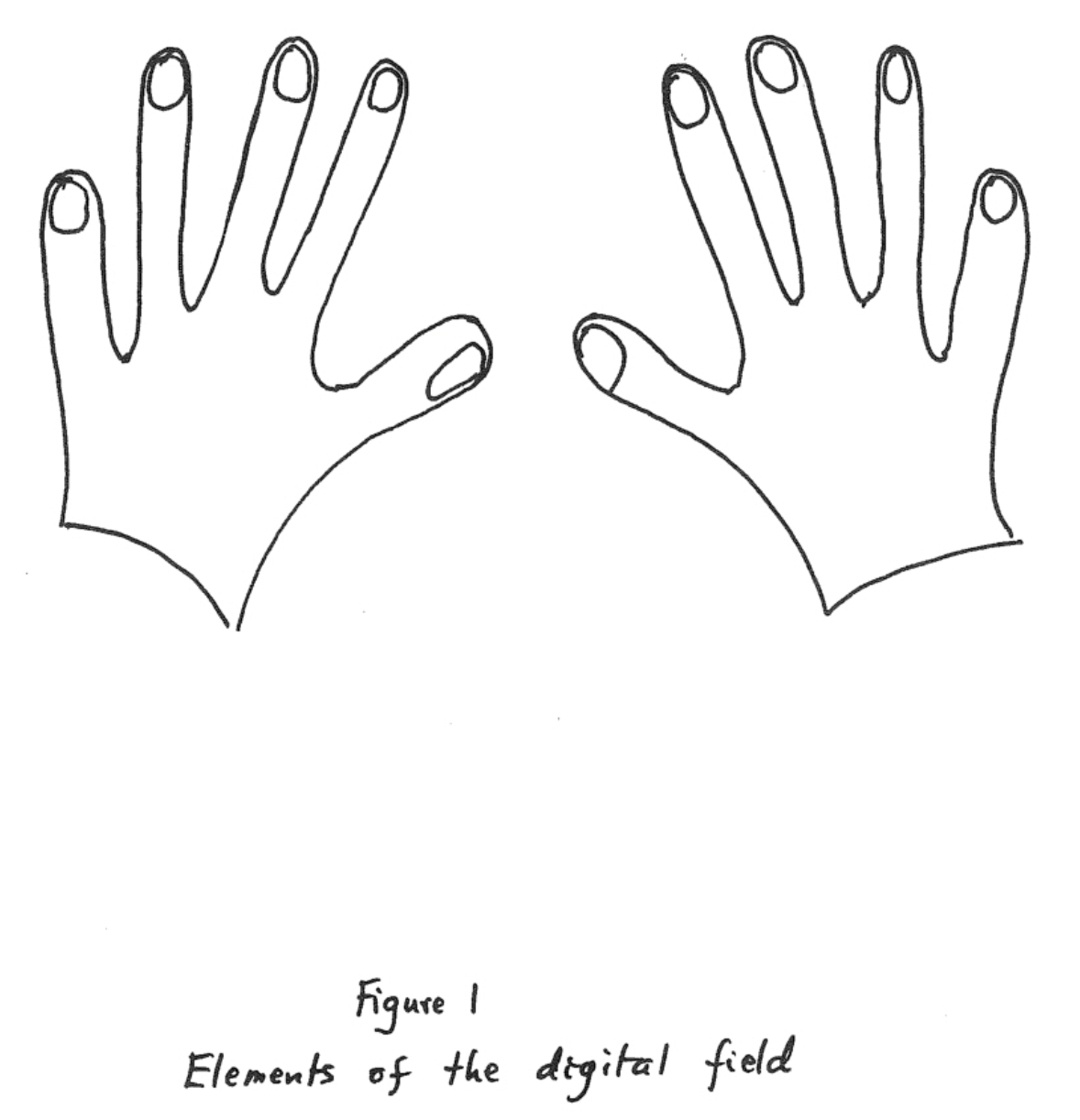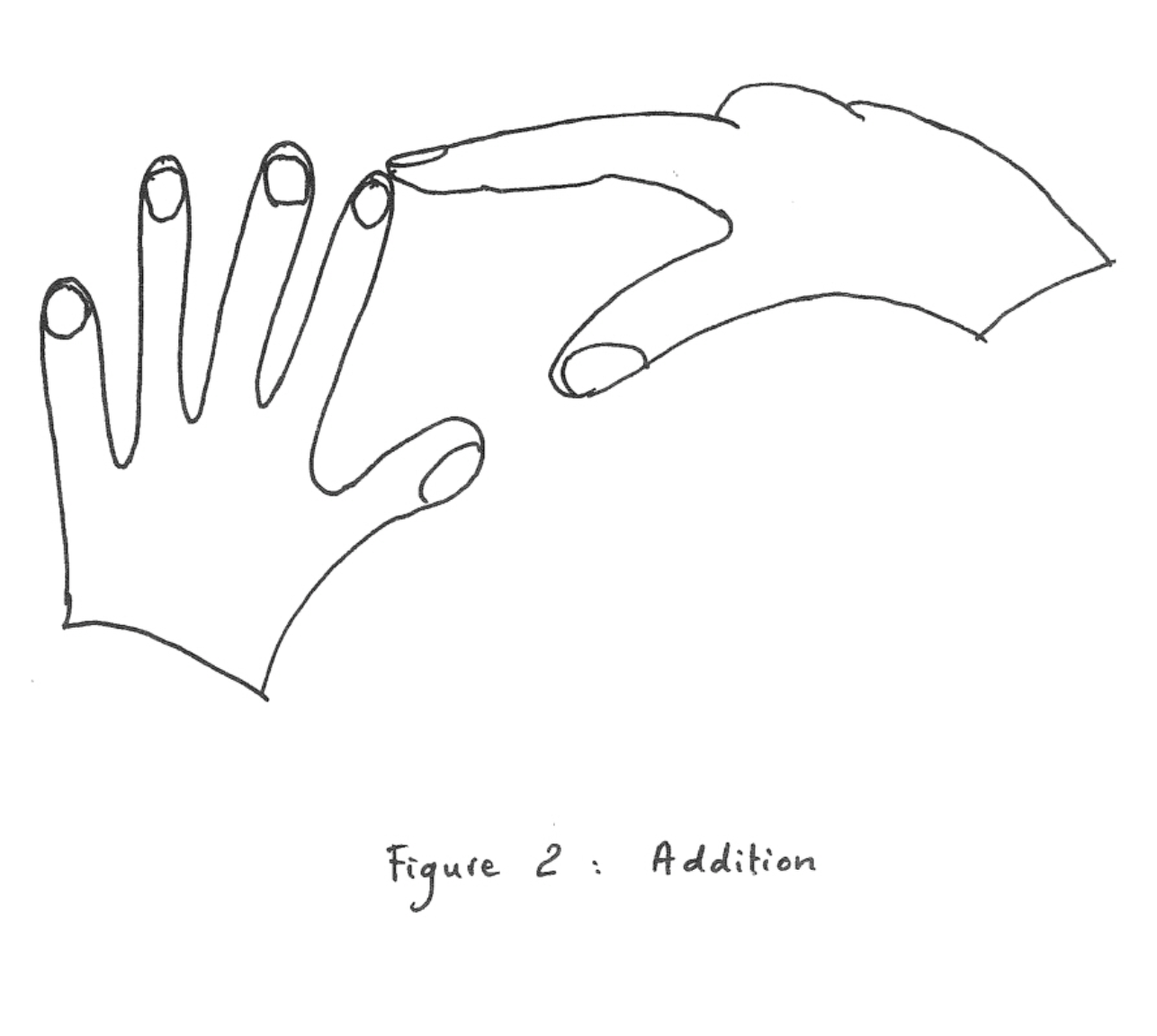Addition is performed by counting, again in the natural way. An example is shown in Fig. 2, and for further details the reader can consult any kindergarten student.

In all digital systems the rules for multiplication can be written down immediately once addition has been defined; for example $2 \times n = n+n$. The reader will easily verify the rest of the details.

Since this field plainly contains ten elements (see Fig. 1) we conclude that there is a projective plain of order ten.

So far, the transcript.

More seriously now, the non-existence of a projective plane of order ten was only established in 1988, heavily depending on computer-calculations. A nice account is given in

Now that recent iPhones nearly have the computing powers of former Cray’s, one might hope for easier proofs.

Fortunately, such a proof now exists, see A SAT-based Resolution of Lam’s Problem by Curtis Bright, Kevin K. H. Cheung, Brett Stevens, Ilias Kotsireas, Vijay Ganesh

David Roberts, aka the HigherGeometer, did a nice post on this
No order-10 projective planes via SAT
.

I’m retiring in two weeks so I’m cleaning out my office.

So far, I got rid of almost all paper-work and have split my book-collection in two: the books I want to take with me, and those anyone can grab away.

Here’s the second batch (math/computer books in the middle, popular science to the right, thrillers to the left).If you’re interested in some of these books (click for a larger image, if you want to zoom in) and are willing to pay the postage, leave a comment and I’ll try to send them if they survive the current ‘take-away’ phase.

Here are two books I definitely want to keep. On the left, an original mimeographed version of Mumford’s ‘Red Book’.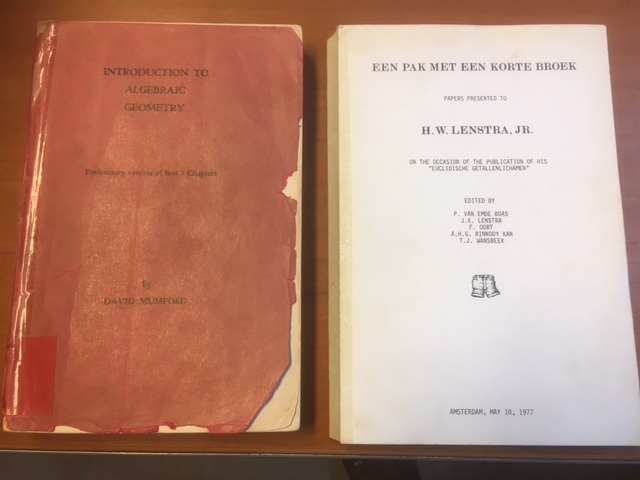On the right, ‘Een pak met een korte broek’ (‘A suit with shorts’), a collection of papers by family and friends, presented to Hendrik Lenstra on the occasion of the defence of his Ph.D. thesis on Euclidean number-fields, May 18th 1977.

If the title intrigues you, a photo of young Hendrik in suit and shorts is included.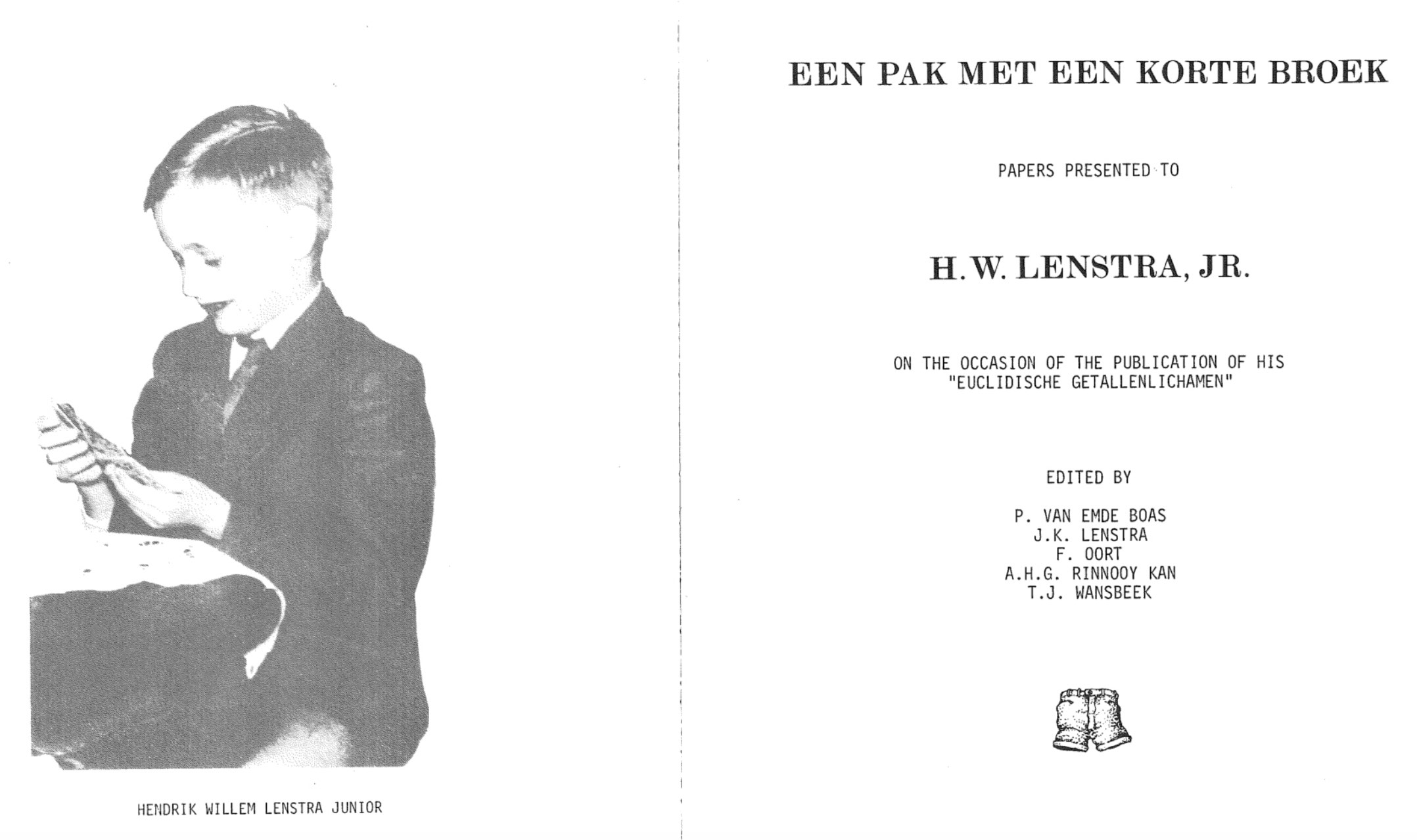This collection includes hilarious ‘papers’ by famous people including

• ‘A headache-causing problem’ by Conway (J.H.), Paterson (M.S.), and Moscow (U.S.S.R.)
• ‘A projective plain of order ten’ by A.M. Odlyzko and N.J.A. Sloane
• ‘La chasse aux anneaux principaux non-Euclidiens dans l’enseignement’ by Pierre Samuel
• ‘On time-like theorems’ by Michiel Hazewinkel
• ‘She loves me, she loves me not’ by Richard K. Guy
• ‘Theta invariants for affine root systems’ by E.J.N. Looijenga
• ‘The prime of primes’ by F. Lenstra and A.J. Oort
• (and many more, most of them in Dutch)

Perhaps I can do a couple of posts on some of these papers. It might break this clean-up routine.

After the death of Grothendieck in November 2014, about 30.000 pages of his writings were found in Lasserre.Since then I’ve been trying to follow what happened to them:

So, what’s new?

In December last year, there was the official opening of the Istituto Grothendieck in the little town of Mondovi in Northern Italy.The videos of the talks given at that meeting are now online.

The Institute houses two centres, the Centre for topos theory and its applications with mission statement:

The Centre for Topoi Theory and its Applications carries out highly innovative research in the field of Grothendieck’s topos theory, oriented towards the development of the unifying role of the concept of topos across different areas of mathematics.

Particularly relevant to these aims is the theory of topos-theoretic ‘bridges’ of Olivia Caramello, coordinator of the Centre and principal investigator of the multi-year project “Topos theory and its applications”.

and the Centre for Grothendiecian studies with mission:

The Centre for Grothendiecian Studies is dedicated to honoring the memory of Alexander Grothendieck through extensive work to valorize his work and disseminate his ideas to the general public.

In particular, the Centre aims to carry out historical/philosophical and editorial work to promote the publication of the unpublished works of A. Grothendieck, as well as to promote the production of translations of already published works in various languages.

No comment on the first. You can look up the Institute’s Governance page, contemplate recent IHES-events, and conjure up your own story.

More interesting is the Centre of Grothendiec(k)ian studies. Here’s the YouTube-clip of the statement made by Johanna Grothendieck (daughter of) at the opening.

She hopes for two things: to find money and interested persons to decrypt and digitalise Grothendieck’s Lasserre gribouillis, and to initiate the re-edition of the complete mathematical works of Grothendieck.

So far, Grothendieck’s family was withholding access to the Lasserre writings. Now they seem to grant access to the Istituto Grothendieck and authorise it to digitalise the 30.000 pages.

Further good news is that a few weeks ago Mateo Carmona was appointed as coordinator of the Centre of grothendieckian studies.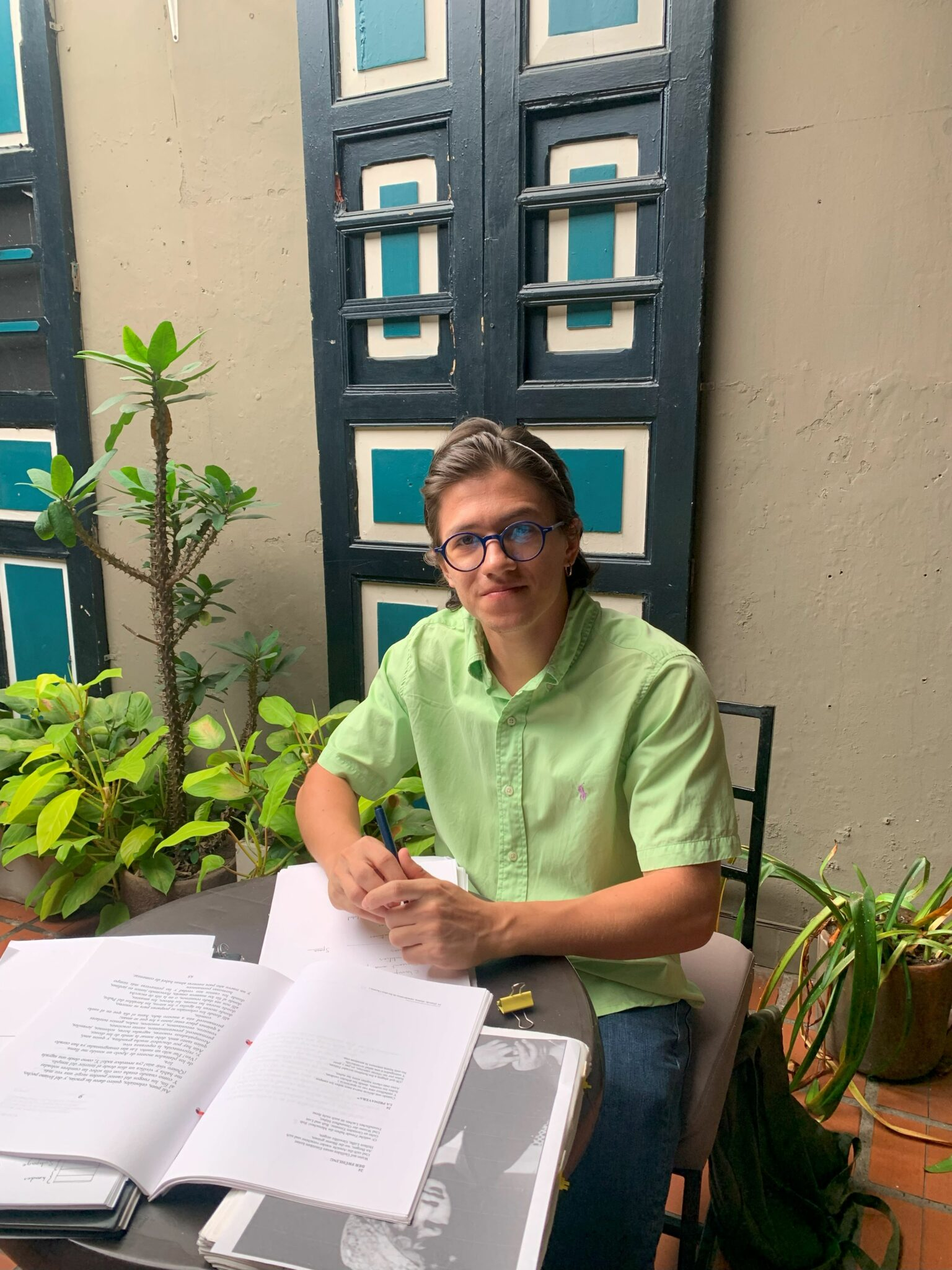You may know Mateo from his Grothendieck Github Archive. A warning note on that page states: “This site no longer updates (since Feb. 2023) and has been archived. Please visit [Instituto Grothendieck] or write to Mateo Carmona at mateo.carmona@csg.igrothendieck.org”. So probably the site will be transferred to the Istituto.

Mateo Carmona says:

As Coordinator of the CSG, I will work tirelessly to ensure that the Centre provides comprehensive resources for scholars, students, and enthusiasts interested in Grothendieck’s original works and modern scholarship. I look forward to using my expertise to coordinate and supervise the work of the international group of researchers and volunteers who will promote Grothendieck’s scientific and cultural heritage through the CSG.

It looks as if Grothendieck’s gribouillis are in good hands, at last.

In a recent post I recalled Claude Levy-Strauss’ observation “In Paris, intellectuals need a new toy every 15 years”, and gave a couple of links showing that the most recent IHES-toy has been spreading to other Parisian intellectual circles in recent years.

At the time (late sixties), Levy-Strauss was criticising the ongoing Foucault-hype. It appears that, since then, the frequency of a hype cycle is getting substantially shorter.

Ten days ago, the IHES announced that Dustin Clausen (of condensed math fame) is now joining the IHES as a permanent professor.

To me, this seems like a sensible decision, moving away from (too?) general topos theory towards explicit examples having potential applications to arithmetic geometry.

On the relation between condensed sets and toposes, here’s Dustin Clausen talking about “Toposes generated by compact projectives, and the example of condensed sets”, at the “Toposes online” conference, organised by Alain Connes, Olivia Caramello and Laurent Lafforgue in 2021.

Two days ago, Clausen gave another interesting (inaugural?) talk at the IHES on “A Conjectural Reciprocity Law for Realizations of Motives”.

We start from a large data-set $V=\{ k,l,m,n,\dots \}$ (texts, events, DNA-samples, …) with a suitable distance-function ($d(m,n) \geq 0~d(k,l)+d(l,m) \geq d(k.m)$) which measures the (dis)similarity between individual samples.

We’re after a set of unknown events $\{ p,q,r,s,\dots \}$ to explain the distances between the observed data. An example: let’s assume we’ve sequenced the DNA of a set of species, and computed a Hamming-like distance to measures the differences between these sequences.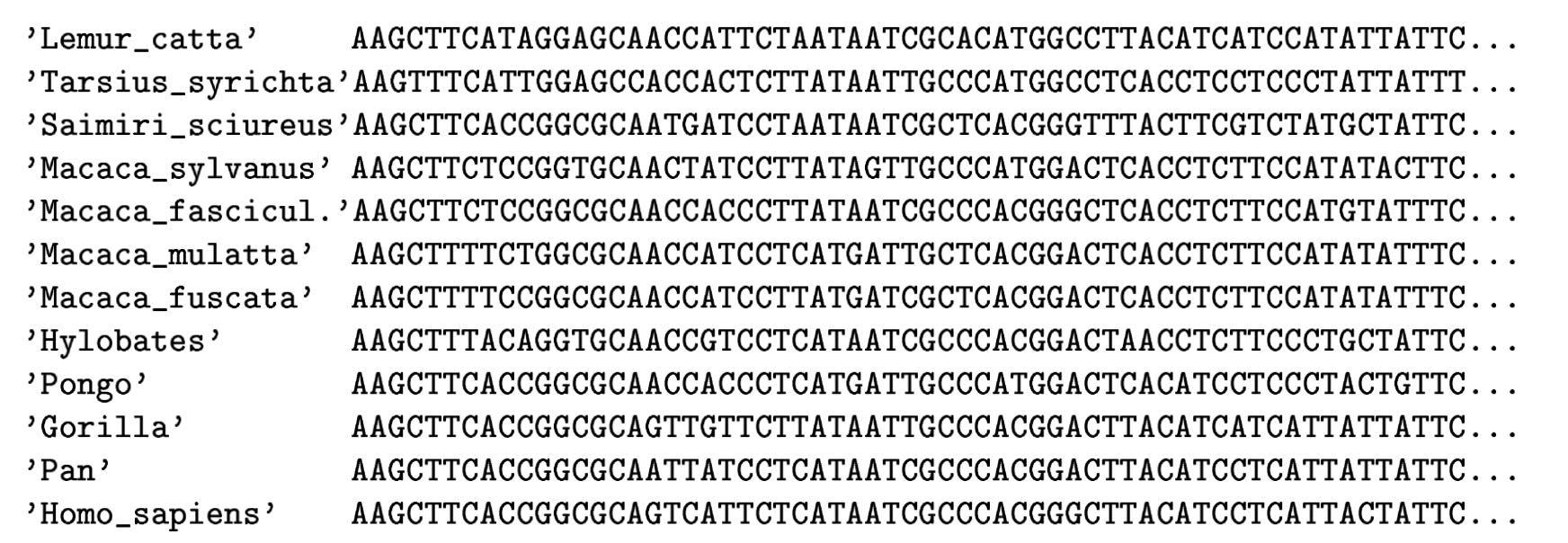(From Geometry of the space of phylogenetic trees by Billera, Holmes and Vogtmann)

Biology explains these differences from the fact that certain species may have had more recent common ancestors than others. Ideally, the measured distances between DNA-samples are a tree metric. That is, if we can determine the full ancestor-tree of these species, there should be numbers between ancestor-nodes (measuring their difference in DNA) such that the distance between two existing species is the sum of distances over the edges of the unique path in this phylogenetic tree connecting the two species.

Last time we’ve see that a necessary and sufficient condition for a tree-metric is that for every quadruple $k,l,m,n \in V$ we have that the maximum of the sum-distances

$$\{ d(k,l)+d(m,n),~d(k,m)+d(l,n),~d(k,n)+d(l,m) \}$$

is attained at least twice.

In practice, it rarely happens that the measured distances between DNA-samples are a perfect fit to this condition, but still we would like to compute the most probable phylogenetic tree. In the above example, there will be two such likely trees: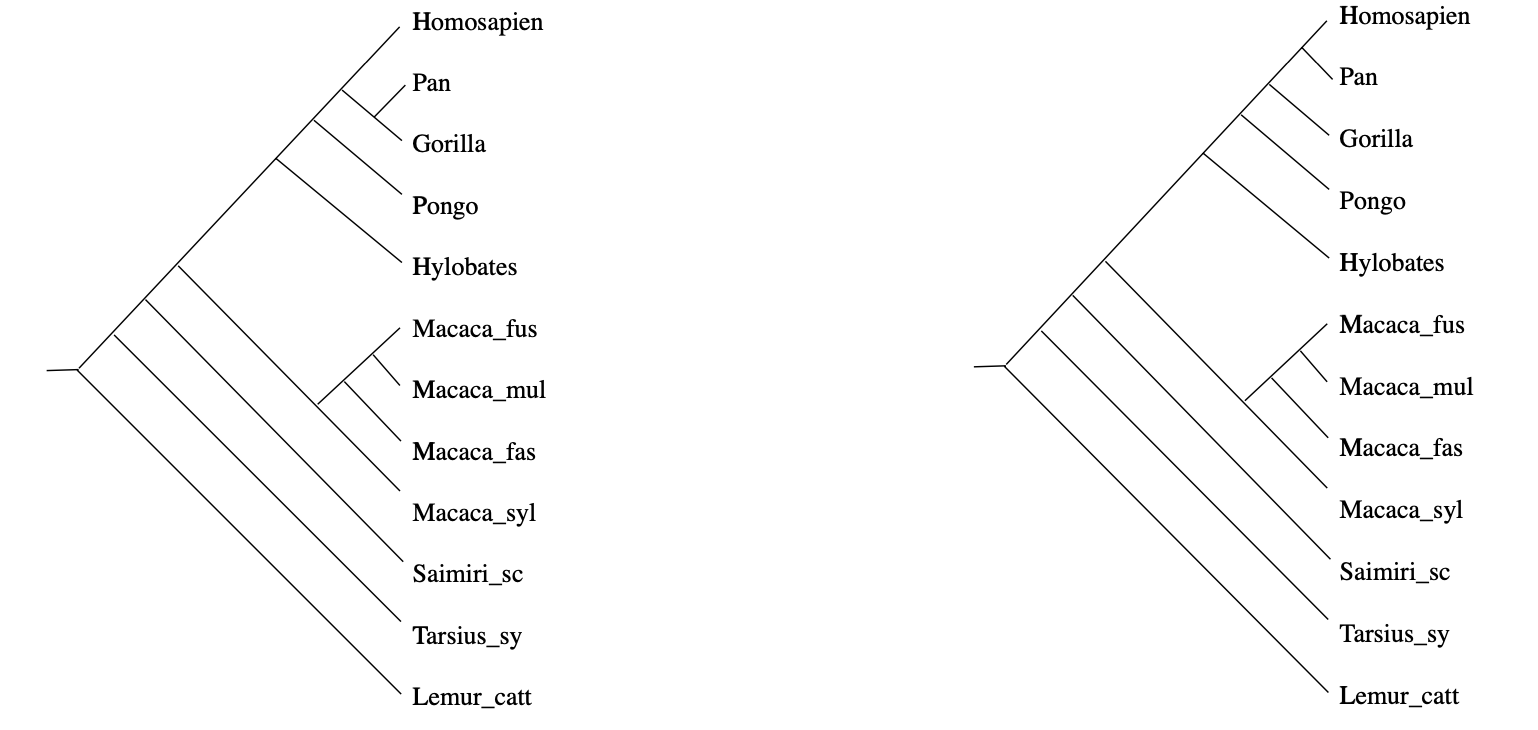(From Geometry of the space of phylogenetic trees by Billera, Holmes and Vogtmann)

How can we find them? And, if the distances in our data-set do not have such a direct biological explanation, is it still possible to find such trees of events (or perhaps, a forest of event-trees) explaining our distance function?

Well, tracking back these ancestor nodes looks a lot like trying to construct colimits.

By now, every child knows that if their toy category $T$ does not allow them to construct all colimits, they can always beg for an upgrade to the presheaf topos $\widehat{T}$ of all contravariant functors from $T$ to $Sets$.

But then, the child can cobble together too many crazy constructions, and the parents have to call in the Grothendieck police who will impose one of their topologies to keep things under control.

Can we fall back on this standard topos philosophy in order to find these forests of the unconscious?(Image credit)

We have a data-set $V$ with a distance function $d$, and it is fashionable to call this setting a $[0,\infty]$-‘enriched’ category. This is a misnomer, there’s not much ‘category’ in a $[0,\infty]$-enriched category. The only way to define an underlying category from it is to turn $V$ into a poset via $n \geq m$ iff $d(n,m)=0$.

Still, we can define the set $\widehat{V}$ of $[0,\infty]$-enriched presheaves, consisting of all maps
$$p : V \rightarrow [0,\infty] \quad \text{satisfying} \quad \forall m,n \in V : d(m,n)+p(n) \geq p(m)$$
which is again a $[0,\infty]$-enriched category with distance function
$$\hat{d}(p,q) = \underset{m \in V}{max} (q(m) \overset{.}{-} p(m)) \quad \text{with} \quad a \overset{.}{-} b = max(a-b,0)$$
so $\widehat{V}$ is a poset via $p \geq q$ iff $\forall m \in V : p(m) \geq q(m)$.

The good news is that $\widehat{V}$ contains all limits and colimits (because $[0,\infty]$ has sup’s and inf’s) and that $V$ embeds isometrically in $\widehat{V}$ via the Yoneda-map
$$m \mapsto y_m \quad \text{with} \quad y_m(n)=d(n,m)$$
The mental picture of a $[0,\infty]$-enriched presheaf $p$ is that of an additional ‘point’ with $p(m)$ the distance from $y_m$ to $p$.

But there’s hardly a subobject classifier to speak of, and so no Grothendieck topologies nor internal logic. So, how can we select from the abundance of enriched presheaves, the nodes of our event-forest?

We can look for special properties of the ancestor-nodes in a phylogenetic tree.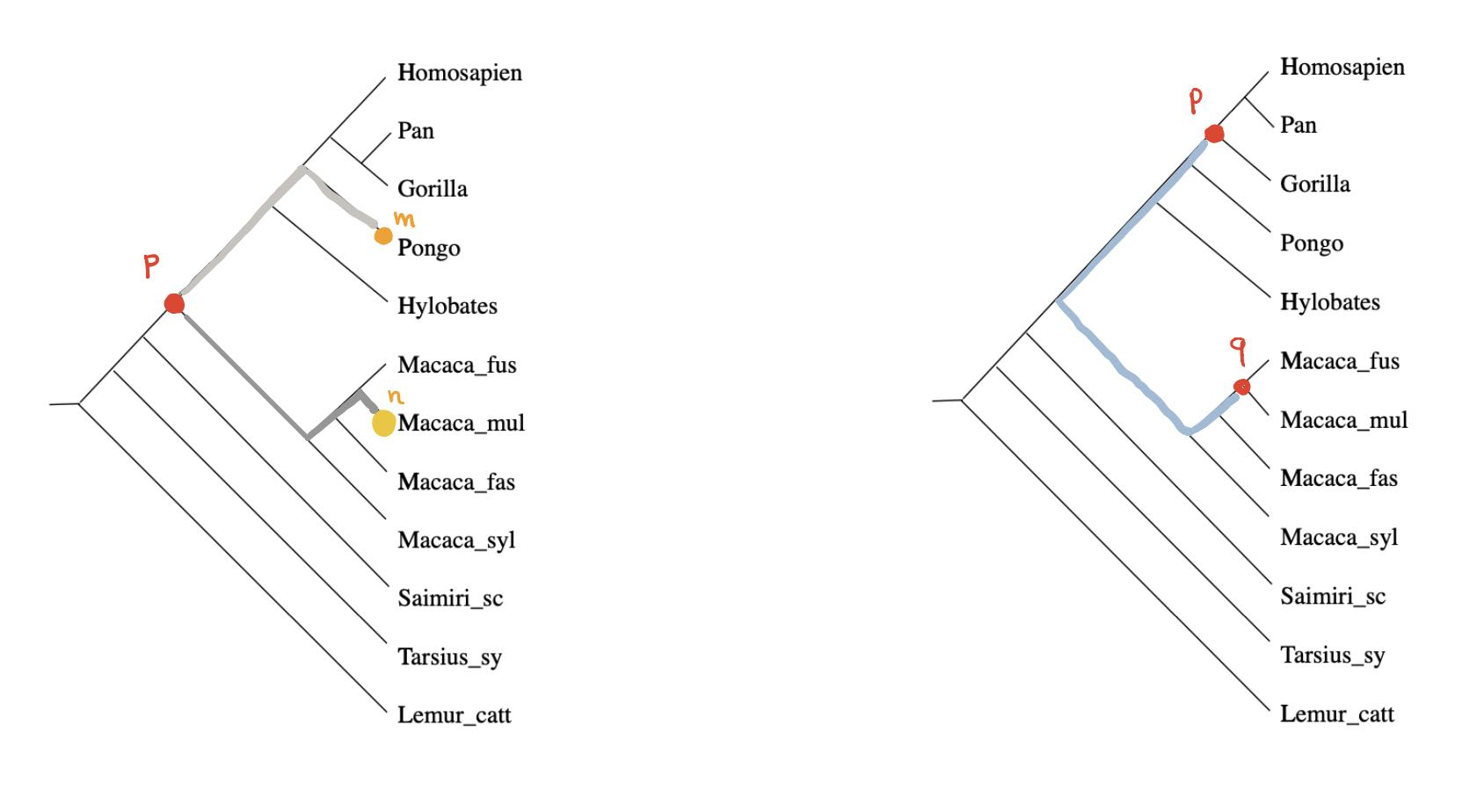For any ancestor node $p$ and any $m \in V$ there is a unique branch from $p$ having $m$ as a leaf (picture above,left). Take another branch in $p$ and a leaf vertex $n$ of it, then the combination of these two paths gives the unique path from $m$ to $n$ in the phylogenetic tree, and therefore
$$\hat{d}(y_m,y_n) = d(m,n) = p(m)+p(n) = \hat{d}(p,y_m) + \hat{d}(p,y_n)$$
In other words, for every $m \in V$ there is another $n \in V$ such that $p$ lies on the geodesic from $m$ to $n$ (identifying elements of $V$ with their Yoneda images in $\widehat{V}$).

Compare this to Stephen Wolfram’s belief that if we looked properly at “what ChatGPT is doing inside, we’d immediately see that ChatGPT is doing something “mathematical-physics-simple” like following geodesics”.

Even if the distance on $V$ is symmetric, the extended distance function on $\widehat{V}$ is usually far from symmetric. But here, as we’re dealing with a tree-distance, we have for all ancestor-nodes $p$ and $q$ that $\hat{d}(p,q)=\hat{d}(q,p)$ as this is just the som of the weights of the edges on the unique path from $p$ and $q$ (picture above, on the right).

Right, now let’s look at a non-tree distance function on $V$, and let’s look at those elements in $\widehat{V}$ having similar properties as the ancestor-nodes:

$$T_V = \{ p \in \widehat{V}~:~\forall n \in V~:~p(n) = \underset{m \in V}{max} (d(m,n) \overset{.}{-} p(m)) \}$$

Then again, for every $p \in T_V$ and every $n \in V$ there is an $m \in V$ such that $p$ lies on a geodesic from $n$ to $m$.

The simplest non-tree example is $V = \{ a,b,c,d \}$ with say

$$d(a,c)+d(b,d) > max(d(a,b)+d(c,d),d(a,d)+d(b,c))$$

In this case, $T_V$ was calculated by Andreas Dress in Trees, Tight Extensions of Metric Spaces, and the Cohomological Dimension of Certain Groups: A Note on Combinatorial Properties of Metric Spaces. Note that Dress writes $mn$ for $d(m,n)$.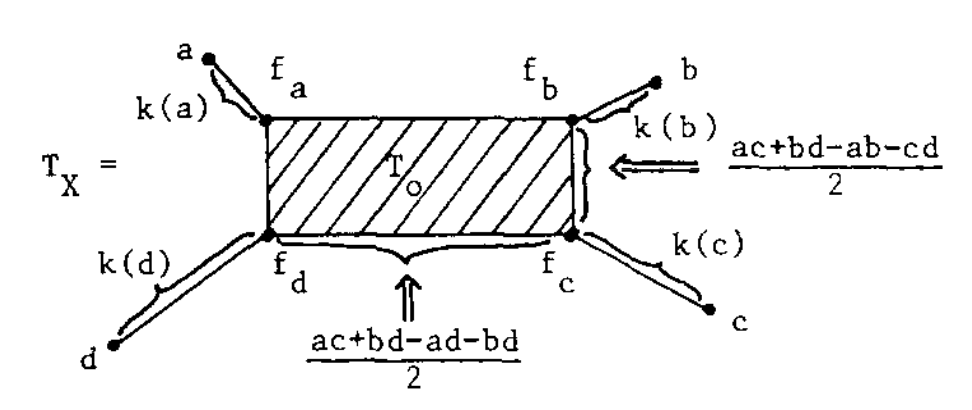If this were a tree-metric, $T_V$ would be the tree, but now we have a $2$-dimensional cell $T_0$ consisting of those presheaves lying on a geodesic between $a$ and $c$, and one between $b$ and $d$. Let’s denote this by $T_0 = \{ a—c,b—d \}$.

$T_V$ has eight $1$-dimensional cells, and with the same notation we have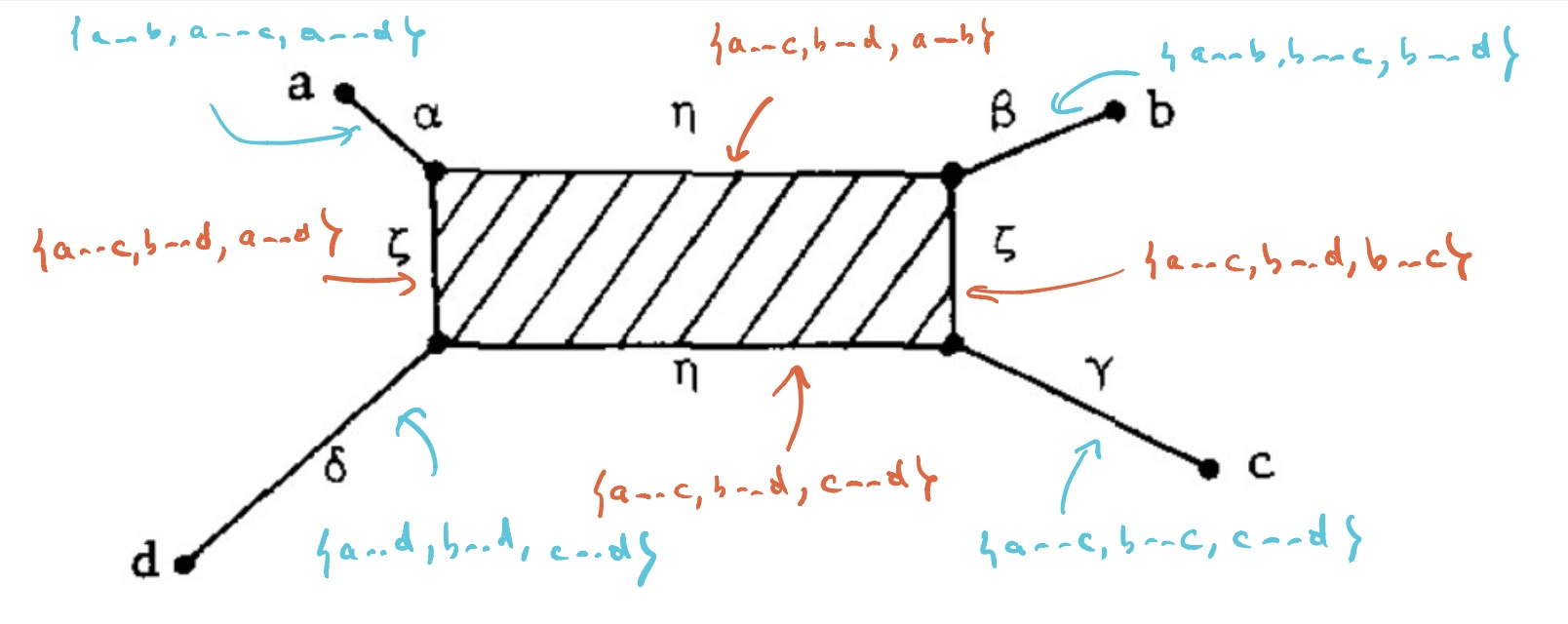Let’s say that $V= \{ a,b,c,d \}$ are four DNA-samples of species but failed to satisfy the tree-metric condition by an error in the measurements, how can we determine likely phylogenetic trees for them? Well, given the shape of the cell-complex $T_V$ there are four spanning trees (with root in $f_a,f_b,f_c$ or $f_d$) having the elements of $V$ as their only leaf-nodes. Which of these is most likely the ancestor-tree will depend on the precise distances.

For an arbitrary data-set $V$, the structure of $T_V$ has been studied extensively, under a variety of names such as ‘Isbell’s injective hull’, ‘tight span’ or ‘tropical convex hull’, in slightly different settings. So, in order to use results one sometimes have to intersect with some (un)bounded polyhedron.

It is known that $T_V$ is always a cell-complex with dimension of the largest cell bounded by half the number of elements of $V$. In this generality it will no longer be the case that there is a rooted spanning tree of teh complex having the elements of $V$ as its only leaves, but we can opt for the best forest of rooted trees in the $1$-skeleton having all of $V$ as their leaf-nodes. Theses are the ‘forests of the unconscious’ explaining the distance function on the data-set $V$.

Apart from the Dress-paper mentioned above, I’ve found these papers informative:

So far, we started from a data-set $V$ with a symmetric distance function, but for applications in LLMs one might want to drop that condition. In that case, Willerton proved that there is a suitable replacement of $T_V$, which is now called the ‘directed tight span’ and which coincides with the Isbell completion.

Recently, Simon Willerton gave a talk at the African Mathematical Seminar called ‘Looking at metric spaces as enriched categories’:

Willerton also posts a series(?) on this at the n-category cafe, starting with Metric spaces as enriched categories I.

(tbc?)

Previously in this series:

On March 20th, David Smith, Joseph Myers, Craig Kaplan and Chaim Goodman-Strauss announced on the arXiv that they’d found an ein-Stein (a stone), that is, one piece to tile the entire plane, in uncountably many different ways, all of them non-periodic (that is, the pattern does not even allow a translation symmetry).

This einStein, called the ‘hat’ (some prefer ‘t-shirt’), has a very simple form : you take the most symmetric of all plane tessellations, $\ast 632$ in Conway’s notation, and glue sixteen copies of its orbifold (or if you so prefer, eight ‘kites’) to form the gray region below: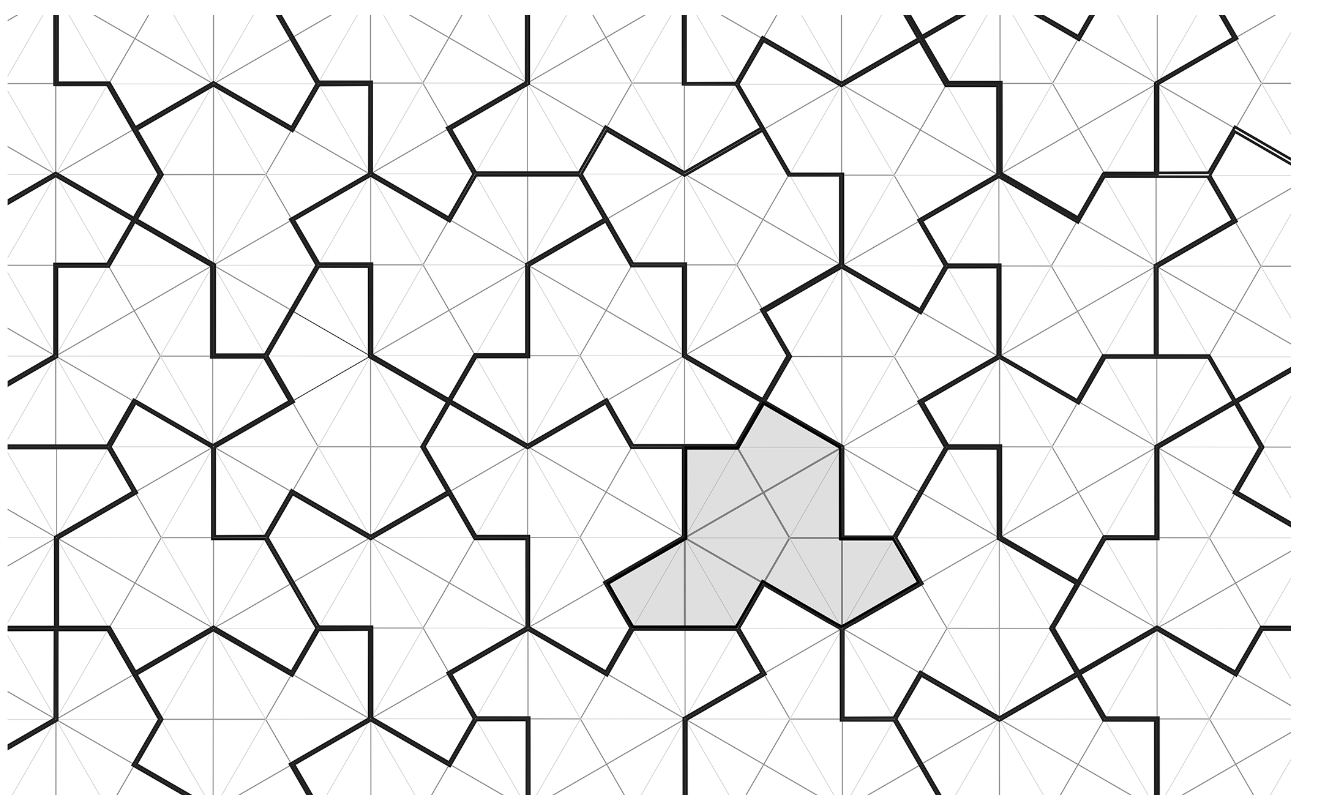(all images copied from the aperiodic monotile paper)

Surprisingly, you do not even need to impose gluing conditions (unlike in the two-piece aperiodic kite and dart Penrose tilings), but you’ll need flipped hats to fill up the gaps left.

A few years ago, I wrote some posts on Penrose tilings, including details on inflation and deflation, aperiodicity, uncountability, Conway worms, and more:

To prove that hats tile the plane, and do so aperiodically, the authors do not apply inflation and deflation directly on the hats, but rather on associated tilings by ‘meta-tiles’ (rough outlines of blocks of hats). To understand these meta-tiles it is best to look at a large patch of hats: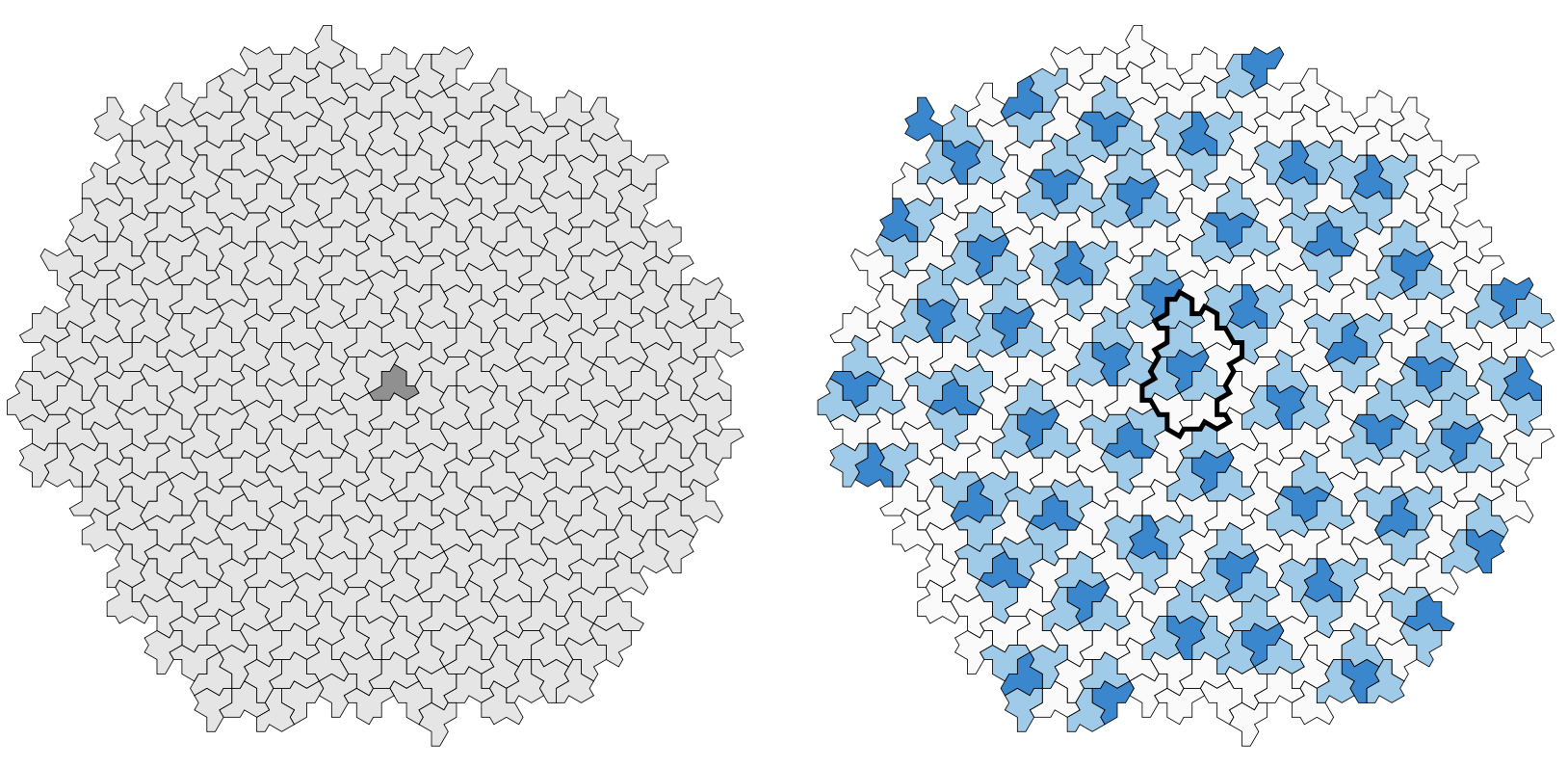Here, the dark-blue hats are the ‘flipped’ ones, and the thickened outline around the central one gives the boundary of the ’empire’ of a flipped hat, that is, the collection of all forced tiles around it. So, around each flipped hat we find such an empire, possibly with different orientation. Also note that most of the white hats (there are also isolated white hats at the centers of triangles of dark-blue hats) make up ‘lines’ similar to the Conway worms in the case of the Penrose tilings. We can break up these ‘worms’ into ‘propeller-blades’ (gray) and ‘parallelograms’ (white). This gives us four types of blocks, the ‘meta-tiles’: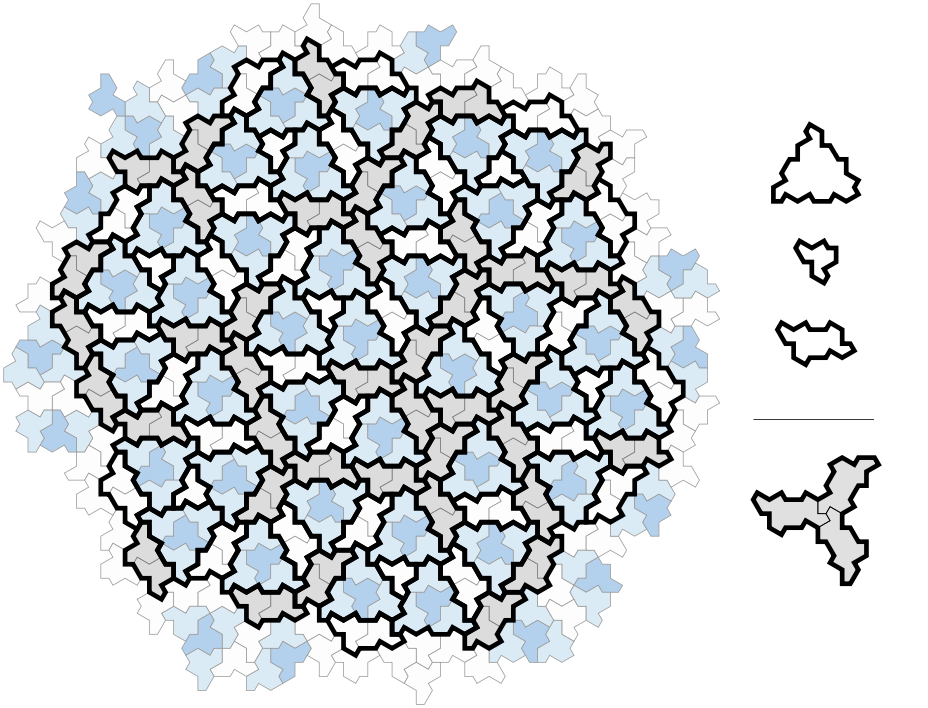The empire of a flipped hat consists of an H-block (for Hexagon) made of one dark-blue (flipped) and three light-blue (ordinary) hats, one P-block (for Parallelogram), one F-block (for Fylfot, a propellor blade), and one T-block (for Triangle) for the remaining hat.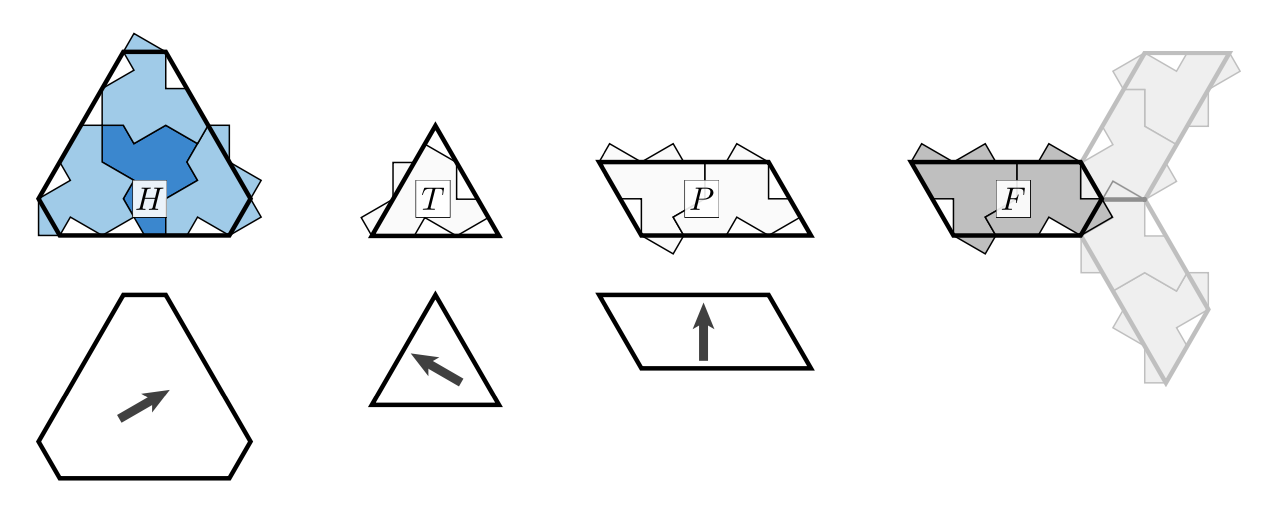The H,T and P blocks have rotational symmetries, whereas the underlying block of hats does not. So we mark the intended orientation of the hats by an arrow, pointing to the side having two or three hat-pieces sticking out.

Any hat-tiling gives us a tiling with the meta-tile pieces H,T,P and F. Conversely, not every tiling by meta-tiles has an underlying hat-tiling, so we have to impose gluing conditions on the H,T,P and F-pieces. We can do this by using the boundary of the underlying hat-block, cutting away and adding hat-parts. Then, any H,T,P and F-tiling satisfying these gluing conditions will come from an underlying hat-tiling.

The idea is now to devise ‘inflation’- and ‘deflation’-rules for the H,T,P and F-pieces. For ‘inflation’ start from a tiling satisfying the gluing (and orientation) conditions, and look for the central points of the propellors (the thick red points in the middle picture).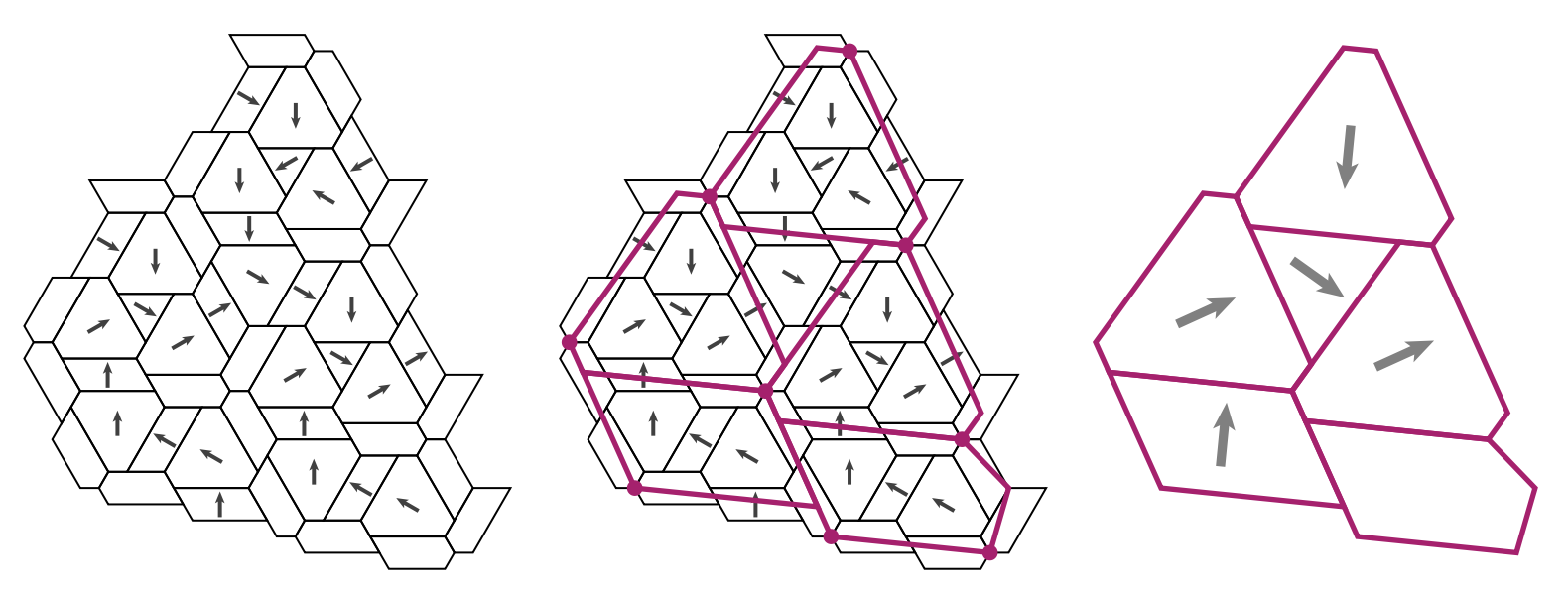These points will determine the shape of the larger H,T,P and F-pieces, together with their orientations. The authors provide an applet to see these inflations in action.

Choose your meta-tile (H,T,P or F), then click on ‘Build Supertiles’ a number of times to get larger and larger tilings, and finally unmark the ‘Draw Supertiles’ button to get a hat-tiling.

For ‘deflation’ we can cut up H,T,P and F-pieces into smaller ones as in the pictures below: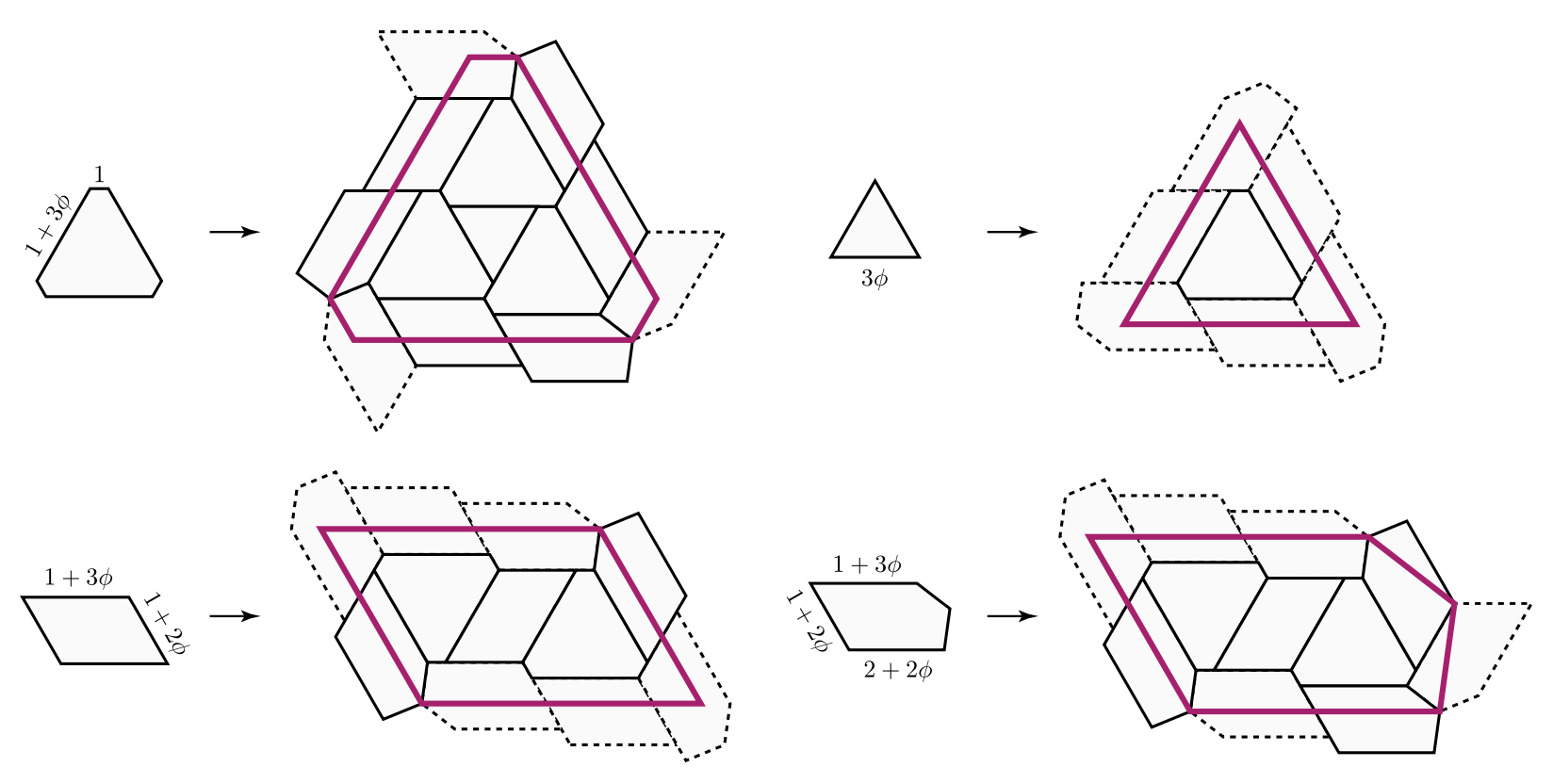Clearly, the hard part is to verify that these ‘inflated’ and ‘deflated’ tilings still satisfy the gluing conditions, so that they will have an underlying hat-tiling with larger (resp. smaller) hats.

This calls for a lengthy case-by-case analysis which is the core-part of the paper and depends on computer-verification.

Once this is verified, aperiodicity follows as in the case of Penrose tilings. Suppose a tiling is preserved under translation by a vector $\vec{v}$. As ‘inflation’ and ‘deflation’ only depend on the direct vicinity of a tile, translation by $\vec{v}$ is also a symmetry of the inflated tiling. Now, iterate this process until the diameter of the large tiles becomes larger than the length of $\vec{v}$ to obtain a contradiction.

Siobhan Roberts wrote a fine article Elusive ‘Einstein’ Solves a Longstanding Math Problem for the NY-times on this einStein.

It would be nice to try this strategy on other symmetric tilings: break the symmetry by gluing together a small number of its orbifolds in such a way that this extended tile (possibly with its reversed image) tile the plane, and find out whether you discovered a new einStein!